# Python Learning Week 7

## Implementation of Greedy Best First Search & A* Search Algorithm

Background

Heuristics can be said to be estimates of how far the goal state is. Heuristics basically predict how far the goal state maybe or how much it will cost to get to the goal state from a particular node.

Greedy Breath First Search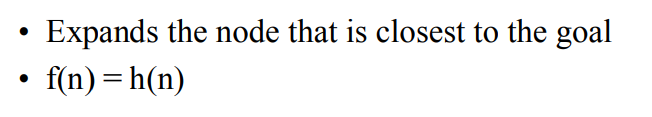Example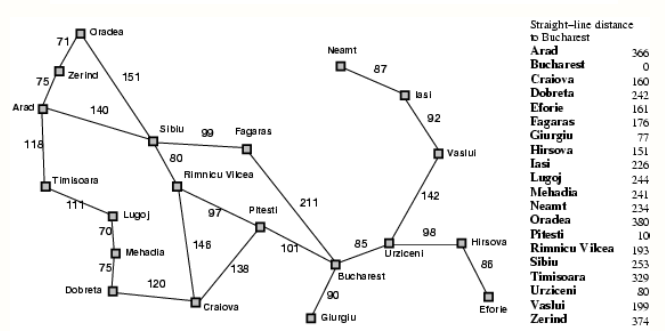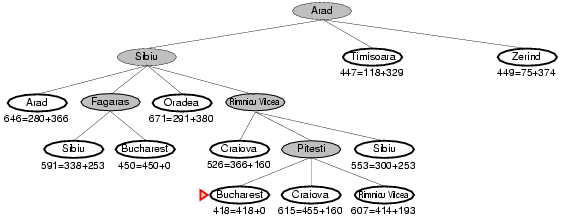Pseudocode (Greedy Breath First Search)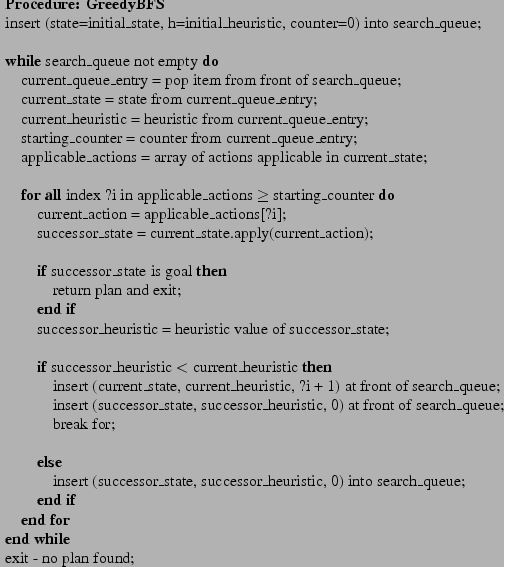A* Algorithm

The A* algorithm combines features of uniform-cost search and pure heuristic search to efficiently compute optimal solutions. A* algorithm is a best-first search algorithm in which the cost associated with a node is f(n) = g(n) + h(n), where g(n) is the cost of the path from the initial state to node n and h(n) is the heuristic estimate or the cost or a path from node n to a goal. Thus, f(n) estimates the lowest total cost of any solution path going through node n. At each point a node with lowest f value is chosen for expansion. Ties among nodes of equal f value should be broken in favor of nodes with lower h values. The algorithm terminates when a goal is chosen for expansion. A* algorithm guides an optimal path to a goal if the heuristic function h(n) is admissible, meaning it never overestimates actual cost. For example, since airline distance never overestimates actual highway distance, and manhatten distance never overestimates actual moves in the gliding tile. For Puzzle, A* algorithm, using these evaluation functions, can find optimal solutions to these problems. In addition, A* makes the most efficient use of the given heuristic function in the following sense: among all shortest-path algorithms using the given heuristic function h(n). A* algorithm expands the fewest number of nodes. The main drawback of A* algorithm and indeed of any best-first search is its memory requirement. Since at least the entire open list must be saved, A* algorithm is severely space-limited in practice, and is no more practical than best-first search algorithm on current machines. For example, while it can be run successfully on the eight puzzle, it exhausts available memory in a matter of minutes on the fifteen puzzle.

Pseudocode (A* Algorithm)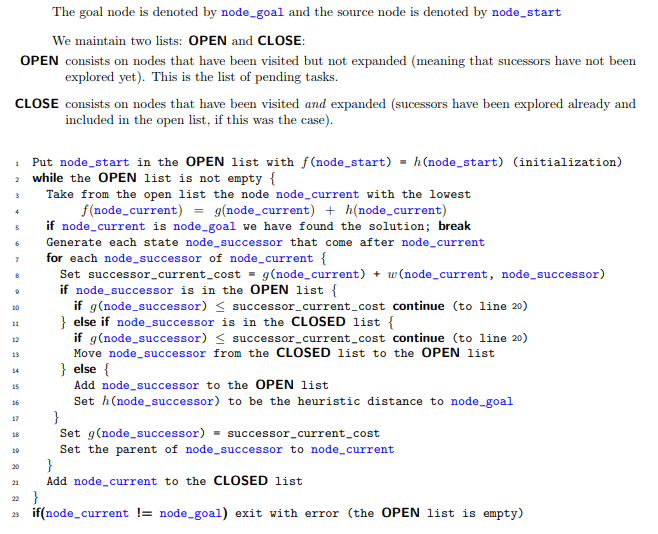Implement Greedy Best First Search Algorithm for the given example, if starting node is Arad and goal node is Bucharest.